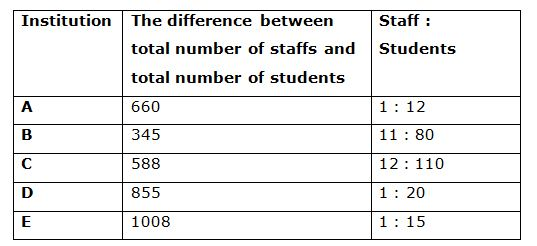# SBI PO Prelims Quantitative Aptitude Questions 2019 – Day 07

SBI PO 2019 Notification will be expected soon. It is one of the most expected recruitment among the banking aspirants. Every year the exam pattern for SBI PO has been changing. Depends upon the changing of exam pattern the questions are quite harder compare to the previous year. So the questions are in high level than the candidate’s assumption.

As per the latest trend, our IBPS Guide is providing the updated New Exam Pattern Quantitative Aptitude questions for SBI PO 2019 Day 7. Our Skilled experts were mounting the questions based on the aspirant’s needs. So candidates shall start your preparation and practice on daily basis with our SBI PO pattern quantitative aptitude questions 2019 day 7. Start your effective preparation from the right beginning to get success in upcoming SBI PO 2019.

[WpProQuiz 5061]

### Click Here for SBI PO Pre 2019 High-Quality Mocks Exactly on SBI Standard

Directions (Q. 1 – 5): In each of these questions two equations (I) and (II) are given. You have to solve both the equations and give answer as,

a) If x > y

b) If x ≥ y

c) If x < y

d) If x ≤ y

e) If x = y or no relation can be established between x and y

1)

I) x2 – 18x + 72 = 0

II) y2 + 2y – 48 = 0

2)

I) 2x2 – 13x + 18 = 0

II) 3y2 + 4y – 15 = 0

3)

I) 4x2 + 14x + 12 = 0

II) 3y2 + 18y + 27 = 0

4)

I) x2 – 4x – 77 = 0

II) y2 + 20y + 64 = 0

5)

I) 3x + 5y = -22

II) 2x – 3y = -2

Directions (Q. 6 – 10) Study the following information carefully and answer the given questions.

The following table shows the difference between total number of staffs and total number of students from various engineering institution and the ratio of staffs to that of Students also given.6) Find the difference between the total number of Staffs in Institution A to that of Institution C?

a) 12

b) 28

c) 34

d) 46

e) None of these

7) Find the ratio between the total number of students in Institution B to that of Institution C?

a) 10: 17

b) 5: 9

c) 33: 59

d) 20: 33

e) None of these

8) Find the average number of Students in Institution A, C and D together?

a) 820

b) 760

c) 650

d) 900

e) None of these

9) Total number of staffs in Institution B is approximately what percentage of total number of staffs in Institution E?

a) 54 %

b) 92 %

c) 76 %

d) 88 %

e) 103 %

10) Find the sum of the total number of staffs in Institution A, B and D together?

a) 160

b) 150

c) 180

d) 170

e) None of these

I) x2 – 18x + 72 = 0

(x – 12) (x – 6) = 0

X = 12, 6

II) y2 + 2y – 48 = 0

(y – 6) (y + 8) = 0

y = 6, -8

X ≥ y

I) 2x2 – 13x + 18 = 0

2x2 – 4x – 9x + 18 = 0

2x (x – 2) -9 (x – 2) = 0

(2x – 9) (x – 2) = 0

X = 9/2, 2 = 4.5, 2

II) 3y2 + 4y – 15 = 0

3y2 + 9y – 5y – 15 = 0

3y (y + 3) – 5 (y + 3) = 0

(3y – 5) (y + 3) = 0

Y = 5/3, -3 = 1.667, -3

X > y

I) 4x2 + 14x + 12 = 0

4x2 + 8x + 6x + 12 = 0

4x (x + 2) + 6(x + 2) = 0

(4x + 6) (x + 2) = 0

X = -6/4, -2 = -1.5, -2

II) 3y2 + 18y + 27 = 0

3y2 + 9y + 9y + 27 = 0

3y (y + 3) + 9 (y + 3) = 0

(3y + 9) (y + 3) = 0

Y = -9/3, -3 = -3, -3

X > y

I) x2 – 4x – 77 = 0

(x – 11) (x + 7) = 0

X = 11, -7

II) y2 + 20y + 64 = 0

(y + 16) (y + 4) = 0

Y = -16, -4

Relationship cannot be determined

3x + 5y = -22 –> (1)

2x – 3y = -2 –> (2)

By solving the equation (1) and (2), we get,

X = -4, y = -2

X < y

Directions (6-10):

The total number of Staffs in Institution A

= > (660/11)*1 = 60

The total number of Staffs in Institution C

= > (588/98)*12 = 72

Required difference = 72 – 60 = 12

The total number of students in Institution B

= > (345/69)*80 = 400

The total number of students in Institution C

= > (588/98)*110 = 660

Required ratio = 400: 660 = 20: 33

The total number of Students in Institution A, C and D together

= > [(660/11)*12] + [(588/98)*110] + [(855/19)*20]

= > 720 + 660 + 900 = 2280

Required average = 2280/3 = 760

Total number of staffs in Institution B

= > (345/69)*11 = 55

Total number of staffs in Institution E

= > (1008/14)*1 = 72

Required % = (55/72)*100 = 76 %